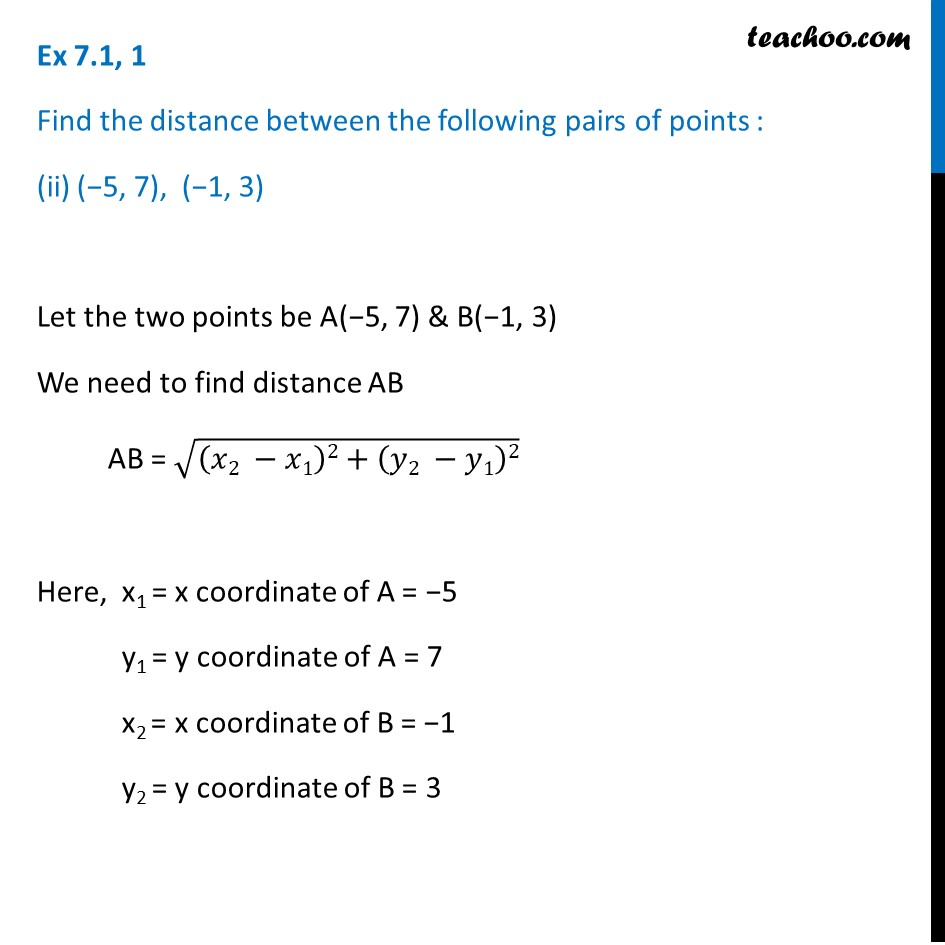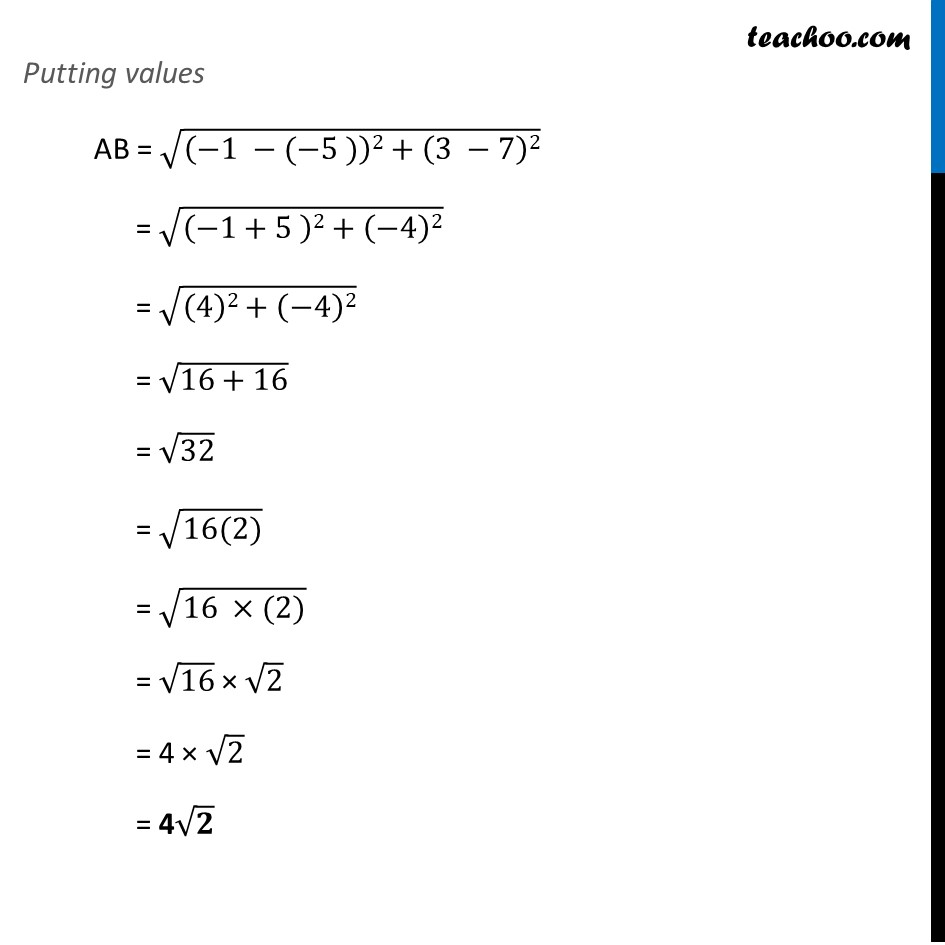Ex 7.1

Chapter 7 Class 10 Coordinate Geometry
Serial order wiseLearn in your speed, with individual attention - Teachoo Maths 1-on-1 Class

### Transcript

Ex 7.1, 1 Find the distance between the following pairs of points : (−5, 7), (−1, 3) Let the two points be A(−5, 7) & B(−1, 3) We need to find distance AB AB = √((𝑥2 −𝑥1)2+(𝑦2 −𝑦1)2) Here, x1 = x coordinate of A = −5 y1 = y coordinate of A = 7 x2 = x coordinate of B = −1 y2 = y coordinate of B = 3 Putting values AB = √((−1 −(−5 ))2+(3 −7)2) = √((−1+5 )2+(−4)2) = √((4)2+(−4)2) = √(16+16) = √32 = √(16(2)) = √(16 ×(2)) = √16 × √2 = 4 × √2 = 4√𝟐